## 5.9Systems of equations

Most CFD calculations involve solving a system of equations that represent the physics of the problem. For example, laminar ﬂow by natural convection can be represented by the equations introduced in Sec. 2.20 , reproduced below.The system provides 3 equations (1 vector, 2 scalar) which can be solved for 3 unknowns,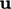,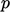and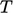.

As discussed in Sec. 3.4 , the ﬁnite volume method solves an individual matrix equation for each variable, e.g.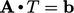for. The vector equation foris decoupled into 3 matrix equations for individual components, i.e.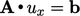,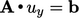and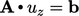.

Each individual matrix equation for one solution variable, e.g.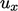, incorporates terms from other variables, e.g.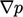, into the source vector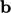. The contribution tois calculated using current values of the respective variables. Systems of equations are thereby solved by successive substitution of solved variables into the source vectors of subsequent equations.

An iterative solution for a single equation, like the one in Sec. 5.7 , can be extended to a system of equations. Timeis incremented by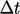and equations are solved in sequence, before returning to start the next time step with the increment of.

The substitutions in the momentum and pressure equations are particularly important, culminating in corrections to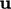and the advective ﬂux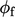, discussed in Sec. 5.10 .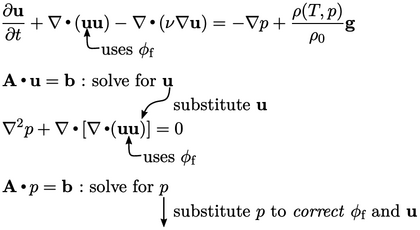At the start of any time step the current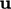becomes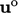for the discretisation of the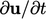term in the momentum equation. The advection term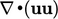is discretised by Eq. (3.8 ), treating oneas ﬂuxand the other as the advected quantity.

The equation is solved for. The new solution foris substituted into the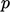equation which is solved for. The new solution foris then used to correct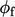in order to help enforce the mass conservation constraint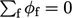(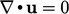).

Before the current solution step is completed,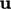is also corrected to reduce the error in the discretisation of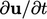when it then becomes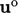in the following solution step. The correction also provides a better “initial guess”for the matrix solution of the next momentum equation, which reduces the solution time.

Notes on CFD: General Principles - 5.9 Systems of equations